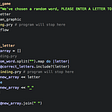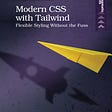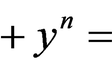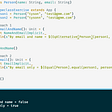# Scala: Either

Why did we need Eithers?
Functional programming strives to have it’s functions total as in for every possible input that the function take, it gives you the correct output that you would expect. However, that is not always possible, and to represent that possibility accurately in the signature, you have Eithers.

What is an Either?
It is a class that represents the two possible outcomes from a computation. These outcomes are not equivalent. The right outcome represents the successful computation of the function, and the left outcome represents the unsuccessful computation of the function.

`Either.cond(true, 100, "failure")`

Eithers are Generics

Different functions are going to have different types for their output types, and also different types to represent their failures. So Eithers are Generic classes and you can specify what the type of your left is, and what the type of your right is.

`Either[Integer,String]`

How would you create an either?

Like this

`Either.cond(true, 100, "failure")`

You specify a predicate condition that evaluates to a boolean, and then you specify the right value which would get returned in the either if the condition evaluated to true, and then you specify the left value which would get returned in the either if the condition evaluated to false.

How would you consume the right value from the either?
You can use the map function like so. The value in the right comes out of the either to be worked with as a raw type.

`Either.cond(true, 100, "failure") .map(right => println(right))`

How would you consume the left value from the either?
You can use left.map function to get the value in the left unwrapped as it’s raw type.

`Either.cond(false, 100, "failure") .map(right => println(right)) .left.map(left => println(left))`

While either is great at representing the two possible states of division of integers, just an integer is easier to deal with than dealing with an either. At some point, you would want to fold the generic either type into a simpler single type. That is where the fold operator comes in and the syntax would be as below.

`val either = Either.cond(true, 100, "failure")println(either.fold(   left => 1000,   right => 2000)+ 1)2001`

The fold method takes two functions, one that transforms the left side of the either to a specific type, and the second one that transforms the right side of the either into the same specific type. Note that the return types of both the functions within the fold method has to be the same.
Also, the return type of the each of the function arguments in the fold method is the return type of the fold method.

Note that the type is no longer an Either after the fold operation. It has become an Int.

Is a fold the same as a combination of .map and .left.map?
It is not. The difference is that .map and .left.map do not change the type of the Either. It is still in either land. Fold basically folded up the either land and packed into it’s own type. Illustrated in a simple example as below.

`val either = Either.cond(true, 100, "failure")println(either.fold(   left => 1000,   right => 2000))println(either.map(_ => 2000)              .left.map(_ => 1000)        )2000Right(2000)`

Swap. One man’s loss is another man’s gain

An Either that represents something on the right and something else on the left can be better represented in some other context with it’s values reversed. For instance if an Either[Int,Int] was representing credit on the right and debit on the left in regards to the money going to John from David, and you had a function that folds the eithers into one financial integer value of how much needs to go to John, and if you now wanted to do the same for David, you could swap the eithers and apply the same function to get the financial integer value that needs to go to David.

`val either = Either.cond(true, 100, "failure")println(either)val eitherSwapped = either.swapprintln(eitherSwapped)Right(100)Left(100)`

Analogous thing would have happened if we had started with a Left instead.

`val either = Either.cond(false, 100, "failure-string")println(either)val eitherSwapped = either.swapprintln(eitherSwapped)Left(failure-string)Right(failure-string)`

I can see the swap as being a useful functionality when you want to look at the same data from a diagonal perspective.

--

--

--

## More from Learn

On a continuing learning journey..

Love podcasts or audiobooks? Learn on the go with our new app.

## Is Serverless Computing the future?## Beginners Guide to Debugging in Ruby## Floats and Clears## Chapter 2 Bootstrapping Your Health## ✍NETWORKING TOPOLOGY SETUP TASK :## 7.4 Pushing Changes## Learn

On a continuing learning journey..

## Scala: Partial functions andThen## Polymorphic functions in Scala## Type Classes in Scala 2